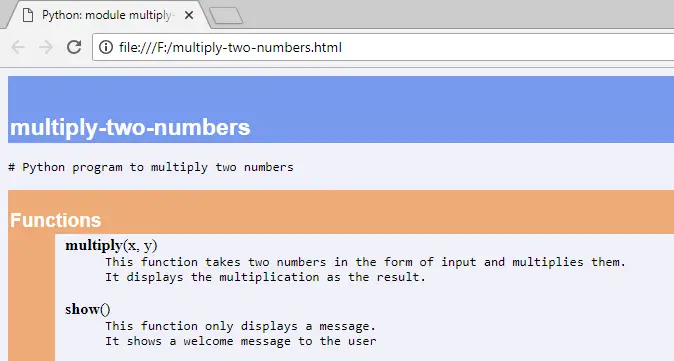## Python Programming Tutorial Index

An API document file is a text or HTML file that contains a description of all the features of the software, language, or product. It creates by a developer, which helps other developers to understand the software and use it correctly. This file also includes details about the classes, modules, functions, etc. used in the software.

In this tutorial, the way to create an API document file in Python is explained through an example.

## Python Program to Multiply Two Numbers

The following Python program includes two functions. The first function is multiplication function that takes two numbers in the form of input and displays their multiplication, and the second function is show function that displays a welcome message to the screen.

Example:

``````# Python program to multiply two numbers
def multiply(x, y):
'''
This function takes two numbers in the form of input and multiplies them.
It displays the multiplication as the result.
'''
print("Result= ",(x+y))

def show():
'''
This function only displays a message.
It shows a welcome message to the user
'''
print("Welcome to Python tutorials.")

# functions calling
multiply(5, 2)
show()``````

Save the above program as the name multiply-two-numbers.py and execute it as:

```F:\>py>python multiply-two-numbers.py
('Result= ', 10)
Welcome to Python tutorials.```
• In the above example program, it used multiply-two-numbers.py as a file name, but you can save as name whatever you want.
• Triple double quote (""") and single quote (''') are called documentation string if these strings are written as first statements in a module, function, class or a method.

This is a standard procedure to execute any Python program. To create an API document, we need to re-execute the program with the Python pydoc module.

Example:

`F:\>py>python -m pydoc -w multiply-two-numbers`

In the above command:

• -m representing a module.
• pydoc is the name of the module.
• -w indicates that an HTML file to be created.
• multiply-to-numbers is a source file name.

The above program displays the output and creates an HTML file named multiply-two-numbers.html.We can see that there is nothing other than the name and description of the two functions written in the program, as mentioned above in the HTML file. In this way, the API documentation represents help on all the features, including functions, classes, modules, etc.